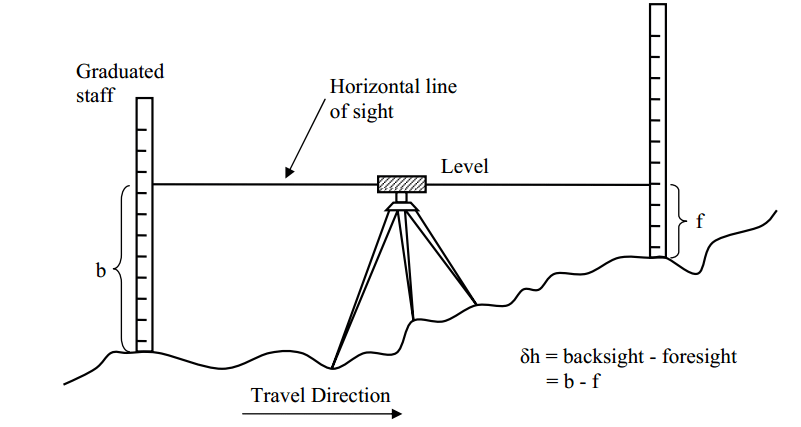# How was mapping before LiDAR?

## Before LiDAR, mapping was done by combining the triangulation and levelling techniques.

### What is triangulation?

Remember your math lessons! The sum of the angles of a triangle equals π radians (180 degrees), the law of sines and the law of cosines, Pythagoras’ theorem, trigonometry… does that mean anything to you?

It’s due to these elements that you can understand triangulation. This mapping technique makes it possible to determine the position of a point in space by measuring its angle as well as that of two other reference points whose position is known. This technique enables precise measurements over large distances, which is not possible by direct measurement of the distance between points.

### What about the levelling techniques?

This technique simply consists of taking heights (altitudes) from a reference point and measuring the deviations to obtain the ground level.Sources : Kemboi, Kiprono & Odera, Patroba. (2016). ESTIMATION OF ORTHOMETRIC HEIGHT USING EGM2008 AND GPS OVER NAIROBI COUNTY AND ITS ENVIRONS. Journal of Agriculture, Science and Technology. 17. 118 – 131.

Later, the technique of photogrammetry helped to make the maps faster. Today, none of these techniques rival the performance of LiDAR.